Next: Impulses Up: Conservation of momentum Previous: Multi-component systems

## Rocket science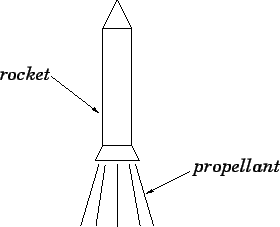A rocket engine is the only type of propulsion device that operates effectively in outer space. As shown in Fig. 50, a rocket works by ejecting a propellant at high velocity from its rear end. The rocket exerts a backward force on the propellant, in order to eject it, and, by Newton's third law, the propellant exerts an equal and opposite force on the rocket, which propels it forward.

Let us attempt to find the equation of motion of a rocket. Let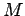be the fixed mass of the rocket engine and the payload, and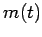the total mass of the propellant contained in the rocket's fuel tanks at time. Suppose that the rocket engine ejects the propellant at some fixed velocityrelative to the rocket. Let us examine the rocket at two closely spaced instances in time. Suppose that at timethe rocket and propellant, whose total mass is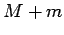, are traveling with instantaneous velocity. Suppose, further, that between timesand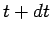the rocket ejects a quantity of propellant of mass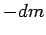(n.b.,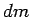is understood to be negative, so this represents a positive mass) which travels with velocity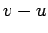(i.e., velocity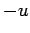in the instantaneous rest frame of the rocket). As a result of the fuel ejection, the velocity of the rocket at timeis boosted to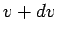, and its total mass becomes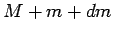. See Fig. 51.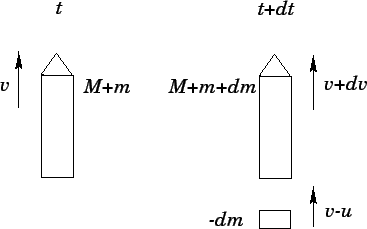Now, there is zero external force acting on the system, since the rocket is assumed to be in outer space. It follows that the total momentum of the system is a constant of the motion. Hence, we can equate the momenta evaluated at timesand: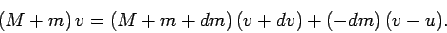(203)

Neglecting second order quantities (i.e.,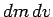), the above expression yields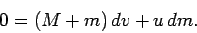(204)

Rearranging, we obtain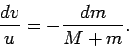(205)

Let us integrate the above equation between an initial time at which the rocket is fully fueled--i.e.,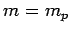, where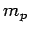is the maximum mass of propellant that the rocket can carry--but stationary, and a final time at which the mass of the fuel isand the velocity of the rocket is. Hence,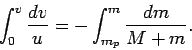(206)

It follows that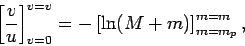(207)

which yields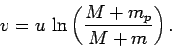(208)

The final velocity of the rocket (i.e., the velocity attained by the time the rocket has exhausted its fuel, so that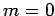) is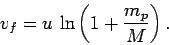(209)

Note that, unless the initial mass of the fuel exceeds the fixed mass of the rocket by many orders of magnitude (which is highly unlikely), the final velocity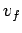of the rocket is similar to the velocitywith which fuel is ejected from the rear of the rocket in its instantaneous rest frame. This follows because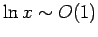, unlessbecomes extremely large.

Let us now consider the factors which might influence the design of a rocket for use in interplanetary or interstellar travel. Since the distances involved in such travel are vast, it is important that the rocket's final velocity be made as large as possible, otherwise the journey is going to take an unacceptably long time. However, as we have just seen, the factor which essentially determines the final velocityof a rocket is the speed of ejectionof the propellant relative to the rocket. Broadly speaking,can never significantly exceed. It follows that a rocket suitable for interplanetary or interstellar travel should have as high an ejection speed as practically possible. Now, ordinary chemical rockets (the kind which powered the Apollo moon program) can develop enormous thrusts, but are limited to ejection velocities below about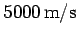. Such rockets are ideal for lifting payloads out of the Earth's gravitational field, but their relatively low ejection velocities render them unsuitable for long distance space travel. A new type of rocket engine, called an ion thruster, is currently under development: ion thrusters operate by accelerating ions electrostatically to great velocities, and then ejecting them. Although ion thrusters only generate very small thrusts, compared to chemical rockets, their much larger ejection velocities (up to 100 times those of chemical rockets) makes them far more suitable for interplanetary or interstellar space travel. The first spacecraft to employ an ion thruster was the Deep Space 1 probe, which was launched from Cape Canaveral on October 24, 1998: this probe successfully encountered the asteroid 9969 Braille in July, 1999.Next: Impulses Up: Conservation of momentum Previous: Multi-component systems
Richard Fitzpatrick 2006-02-02